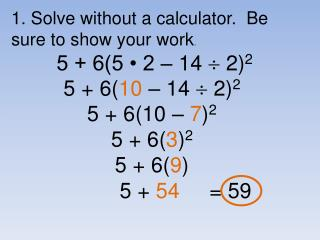DownloadDownload Presentation5 + 6( 10 – 14  2) 2 5 + 6(10 – 7 ) 2 5 + 6( 3 ) 2 5 + 6( 9 ) 5 + 54 = 59

# 5 + 6( 10 – 14  2) 2 5 + 6(10 – 7 ) 2 5 + 6( 3 ) 2 5 + 6( 9 ) 5 + 54 = 59

Télécharger la présentation## 5 + 6( 10 – 14  2) 2 5 + 6(10 – 7 ) 2 5 + 6( 3 ) 2 5 + 6( 9 ) 5 + 54 = 59

- - - - - - - - - - - - - - - - - - - - - - - - - - - E N D - - - - - - - - - - - - - - - - - - - - - - - - - - -
##### Presentation Transcript

1. 1. Solve without a calculator. Be sure to show your work. 5 + 6(5 • 2 – 14  2)2 5 + 6(10 – 14  2)2 5 + 6(10 – 7)2 5 + 6(3)2 5 + 6(9) 5 + 54 = 59

2. 1 2 1 + 2 = 180 10x – 10 + 5x + 10 = 180 15x = 180 x = 12 m1 = 10x – 10 = 10(12) – 10 = 110 2. a) What do you call 1 & 2? a linear pair b) if 1 = 10x – 10, 2 = 5x + 10, Find m 1.

3. A B D C 2 1 3 4 F E 1 BCF FCB FCA NOT A! 3. Give another name for: a) CA CB b) AB AC, or AE c) DC CD d) DC CD, DF, CF, FD, FC e) ACF

4. A B D C 2 1 3 4 F E 4. Name: a) a pair of vertical angles 1 & 3 2 & 4 b) a linear pair 1 & 4 1 & 2 3 & 4 3 & 2 c) 3 collinear points D,C,F A,B,C A,B,E A,C,E B,C,E

5. 5. 1 is acute. Find the restrictions on x. 6x - 12 1 0 < 6x – 12 < 90 12 < 6x < 102 2 < x < 17

6. 2 1 3 4 1 = 3 7x + 7 = 10x – 2 9 = 3x 3 = x m 1 = 7x + 7 = 7(3) + 7 = 28 1 = 7x + 7, 3 = 10x – 2 , Find m 1.

7. 2 1 3 4 7. If m2 = 98, a) is 1 right, acute, or obtuse? (1 & 2 are a linear pair, so 1 = 82), acute b) is 3 right, acute or obtuse? (2 & 3 are a linear pair, so 3 = 82), acute c) is 4 right, acute or obtuse? (2 & 4 are vertical angles, so 4 = 98),obtuse

8. R K M 8. K is the midpoint of RM RK = 3x + 1, KM = 5x – 15 , Find RM. RK = KM 3x + 1 = 5x – 15 16 = 2x 8 = x RM = 3x + 1 + 5x – 15 = 3(8) +1 + 5(8) – 15 = 50